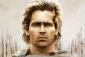# differentiation of transcendental functions

4 posts / 0 new
christian10_mat...
differentiation of transcendental functions

Evaluate the limits
1. lim sin3x/sin2x
X-0

2. lim 1-cosx/4x^2
X-0

thank u

Alexander1. 3/2
2. 1/8

christian10_mat...

Sir can u show the solution. .

AlexanderI am not used to type an equation but here it is.
1. d(sin(3x)) / d(sin(2x)) = 3 cos(3x) / 2 cos(2x). For x=0, cos 0=1., so 3/2
2. d(1-cos x) / d(4x^2) = sinx/8x = d(sinx)/d(8x) = cosx/8. For x=0, 1/8

• Mathematics inside the configured delimiters is rendered by MathJax. The default math delimiters are $$...$$ and $...$ for displayed mathematics, and $...$ and $...$ for in-line mathematics.